# Electrostatics Magnetic Effect of Current, NCERT Physics Class 12, MCQ Quiz Test

#### How to Count Your Score

You will get 1 point for each correct answer. At the end of the Quiz, your total score will be displayed. Maximum score is 25 points.

Exam is Over

MCQ 1:
The wire loop formed by joining two semicircular sections of radii R1 and R2, and centre C, carries a current I, as shown. The magnetic field at C has magnitude –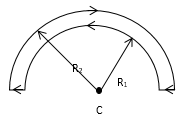MCQ 2:
B along the axis of a solenoid is given by –

MCQ 3:
Magnetic field is not associated with

MCQ 4:
A current-carrying, straight wire is kept along the axis of a circular loop carrying a current. The straight wire:

MCQ 5:
A vertical wire carries a current in upward direction. An electron beam sent horizontally towards the wire will be deflected

MCQ 6:
If the electric field is uniform, then the electric lines of forces are -

MCQ 7:
Electric lines of forces-

MCQ 8:
Which one of the following diagrams shows the correct lines of force ?

MCQ 9:
In fig. shown the electric lines of force emerging from a charged body. If the electric fields at A and B are EA and EB are respectively and the distance between A and B is r then -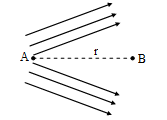MCQ 10:
A metallic solid sphere is placed in a uniform electric field. The lines of force follow the path(s) shown in figure as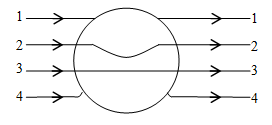MCQ 11:
Three positive charges of equal value q are placed at the vertices of an equilateral triangle. The resulting lines of force should be sketched as in –

MCQ 12:
A point charge ‘q’ is placed at a point inside a hollow conducting sphere which of the following electric lines of force pattern is correct ?

MCQ 13:
A few electric field lines for a system of two charges Q1 and Q2 fixed at two different points on the x-axis are shown in the figure. These lines suggest that -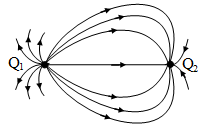MCQ 14:
Fg and Fe represent the gravitational and electrostatic force respectively between two protons situated at some distance in vacuum. The ratio of Fg to Fe is of the order of

MCQ 15:
When the distance between two charged particle is halved, the force between them becomes -

MCQ 16:
A certain charge Q is divided at first into two parts, (q) and (Q–q). Later on the charges are placed at a certain distance. If the force of interaction between the two charges is maximum then-

MCQ 17:
Three equal charges (q) are placed at corners of a equilateral triangle. The force on any charge is -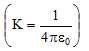MCQ 18:
Two charges 9e and 3e are placed at a distance r. The distance of the point where the electric field intensity will be zero is -

MCQ 19:
Six charges +Q each are placed at the corners of a regular hexagon of side (a), the electric field at the centre of hexagon is-

MCQ 20:
A uniformly charged rod with charge per unit length λ is bent in to the shape of a semicircle of radius R. The electric field at the centre is -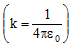MCQ 21:
If an electric dipole is kept in a uniform electric field. Then it will experience-

MCQ 22:
An electric dipole consists of two opposite charges each of magnitude 1 × 10–6 C separated by a distance 2 cm. The dipole is placed in an external field of 10 × 105 N/C. The maximum torque on the dipole is -

MCQ 23:
The ratio of the electric field due to an electric dipole on its axis and on the perpendicular bisector of the dipole is-

MCQ 24:
A hemisphere (radius R) is placed in electric field as shown in fig. Total outgoing flux is -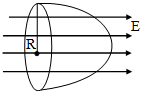MCQ 25:
Two large metal plates each of area (A) carry charges +q and -q and facing each other. The plates are separated by a small distance (d) the electric field between the plates would be -